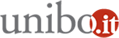# Statistics seminar 2018: Asymptotic Theory for Rotated Multivariate GARCH Models

08/11/2018 dalle 16:00 alle 18:00

Dove Dipartimento di Scienze Statistiche - via delle Belle Arti 41 - Aula SeminariAggiungi l'evento al calendario

Relatore
Michael McAleer
Asia University, Taiwan
Department of Finance

Abstract
In this paper, we derive the statistical properties of a two step approach to estimating multivariate GARCH rotated BEKK (RBEKK) models. By the de_nition of rotated BEKK, we estimate the unconditional covariance matrix in the _rst step in order to rotate observed variables to have the identity matrix for its sample covariance matrix. In the second step, we estimate the remaining parameters via maximizing the quasi-likelihood function. For this two step quasi-maximum likelihood (2sQML) estimator, we show consistency and asymptotic normality under weak conditions. While second-order moments are needed for consistency of the estimated unconditional covariance matrix, the existence of _nite sixth-order moments are required for convergence of the second-order derivatives of the quasi-log-likelihood function.
We also show the relationship of the asymptotic distributions of the 2sQML estimator for the RBEKK model and the variance targeting (VT) QML estimator for the VT-BEKK model.
Monte Carlo experiments show that the bias of the 2sQML estimator is negligible, and that the appropriateness of the diagonal speci_cation depends on the closeness to either of the Diagonal BEKK and the Diagonal RBEKK models.

Organizzazione
Giuseppe Cavaliere
Alessandra Luati